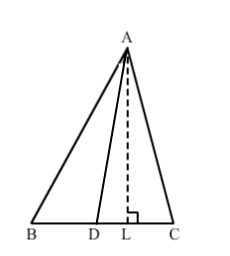# Prove that a median divides a triangle into two triangles of equal area.

Question:

Prove that a median divides a triangle into two triangles of equal area.

Solution:Let AD is a median of ABC and D is the midpoint of BC. AD divides ∆ABC in two triangles: ∆ABD and ADC.
Construction: Draw AL ⊥ BC.
Proof:
Since D is the midpoint of BC, we have:
BD = DC

Multiplying with $\frac{1}{2} A L$ on both sides, we get:

$\frac{1}{2} \times B D \times A L=\frac{1}{2} \times D C \times A L$